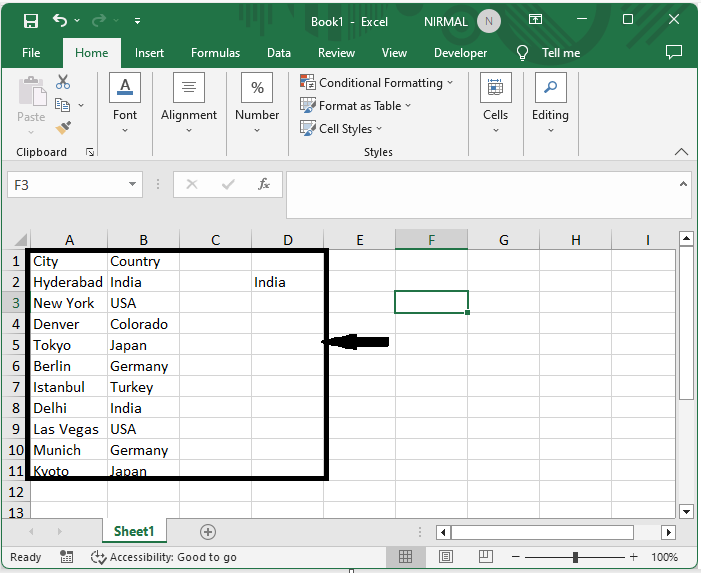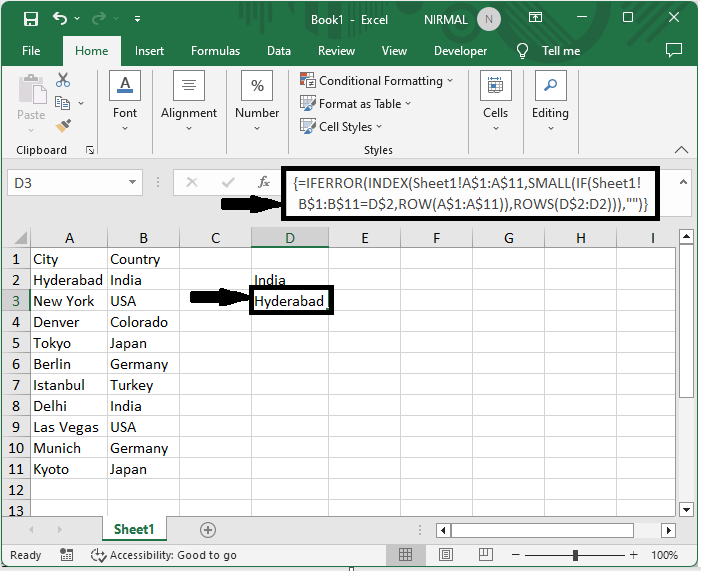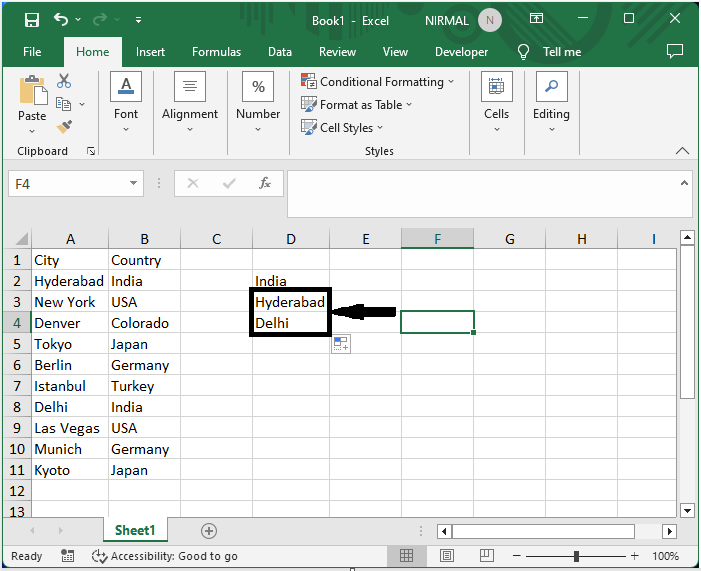# How to Populate Rows Based on Specified Cell Value in Excel?

With the help of Microsoft Excel, users may quickly organise and modify data. Filling in rows based on a defined cell value is a frequent Excel activity. When you need to fill out repeating data or make computations based on a certain situation, this technique is quite helpful. You may save time and guarantee data entry accuracy by automating this process.

## Populate Rows Based on Specified Cell Value

Here we will first get any one of the values using the formula, then use the autofill handle to complete the task. So let us see a simple process to know how you can populate rows based on specified cell values in Excel.

### Step 1

Consider an Excel sheet where the data in the sheet is similar to the below image.First, click on an empty cell in the case of cell E2 and enter the formula as =IFERROR(INDEX(Sheet2!A$1:A$10,SMALL(IF(Sheet2!B$1:B$10=D$2,ROW(A$1:A$10)), ROWS(D$2:D2))),"") and click Ctrl + Shift + Enter to get the first value.

Empty Cell > Formula > Ctrl + Shift + Enter.### Step 2

Then drag down using the autofill handle to complete the task.This is how you can populate rows based on a specified cell value in Excel.

## Conclusion

In this tutorial, we have used a simple example to demonstrate how you can populate rows based on specified cell values in Excel to highlight a particular set of data.

Updated on: 07-Sep-2023

279 Views